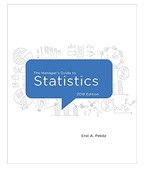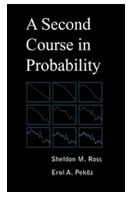Books by Erol A. Peközis an introduction to statistics for aspiring managers, leaders and decision makers who do not need to know all the details of statistical theory and just want real applications and commonsense explanations using words and diagrams -- without a jumble of Greek letters and mathematical formulas. The focus is on teaching and testing intuitive understanding of statistical concepts with applications. This textbook is for an undergraduate or MBA introductory statistics course and covers summary statistics, probability, hypothesis testing, correlation, multiple regression, and includes custom Excel tools for histograms and stepwise regression. You may download the data sets mentioned in the book.  If you are an instructor you can request an evaluation copy and detailed problem solutions by sending an email with your address to pekoz at bu dot edu.A Second Course in Probability is for undergraduate and graduate students in statistics, mathematics, engineering, finance, and actuarial science, and is a guided tour designed to give familiarity with advanced topics in probability without having to wade through the exhaustive coverage of the classic advanced probability theory books.  Topics covered here include measure theory, limit theorems, bounding probabilities and expectations, coupling and Stein's method, martingales, Markov chains, renewal theory, and Brownian motion. No other text covers all these advanced topics rigorously but at such an accessible level; all you need to get started here is calculus and material from a first undergraduate course in probability.  Any other concepts required, such as the definition of convergence, the Lebesgue integral, and of countable and uncountable sets, are introduced as needed.  If you are an instructor you can request an evaluation copy and detailed problem solutions by sending an email with your address to pekoz at bu dot edu.